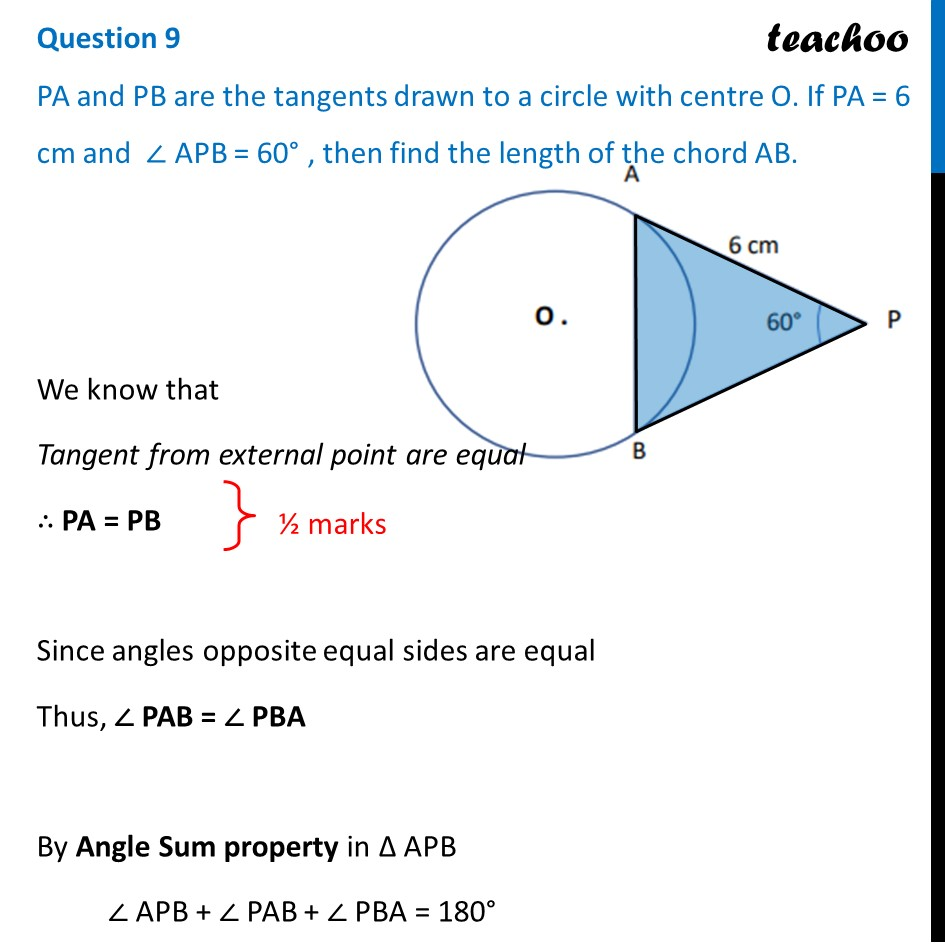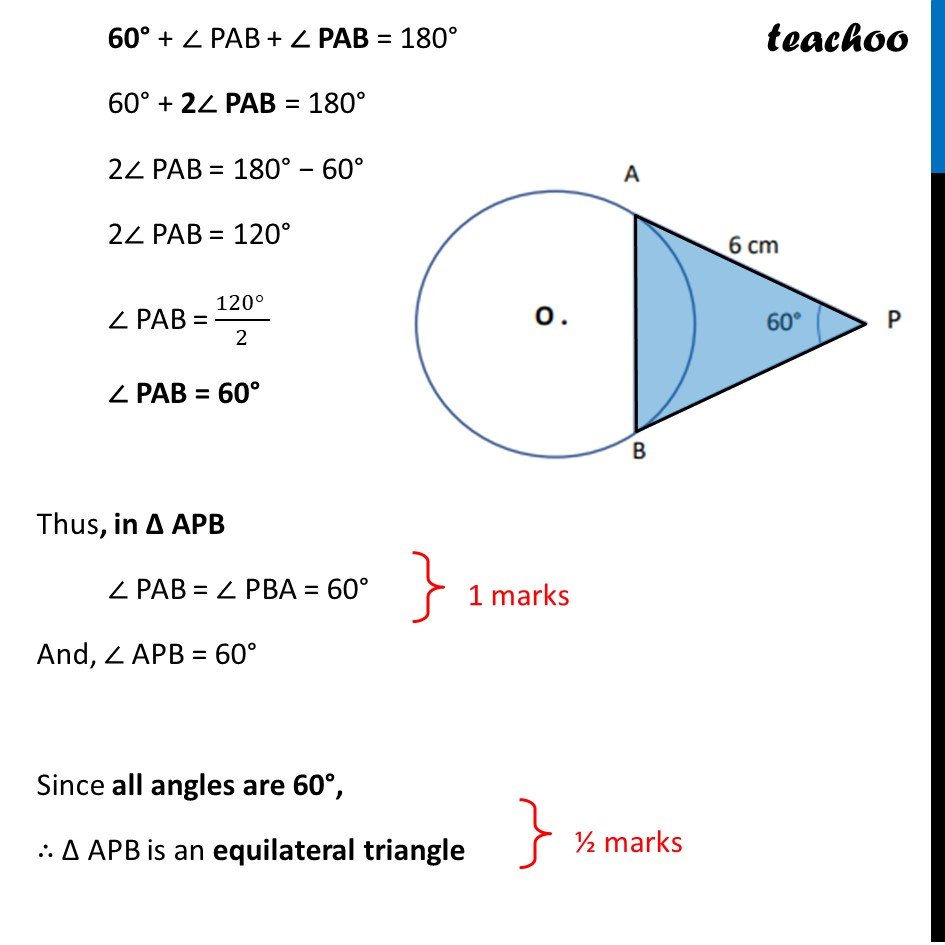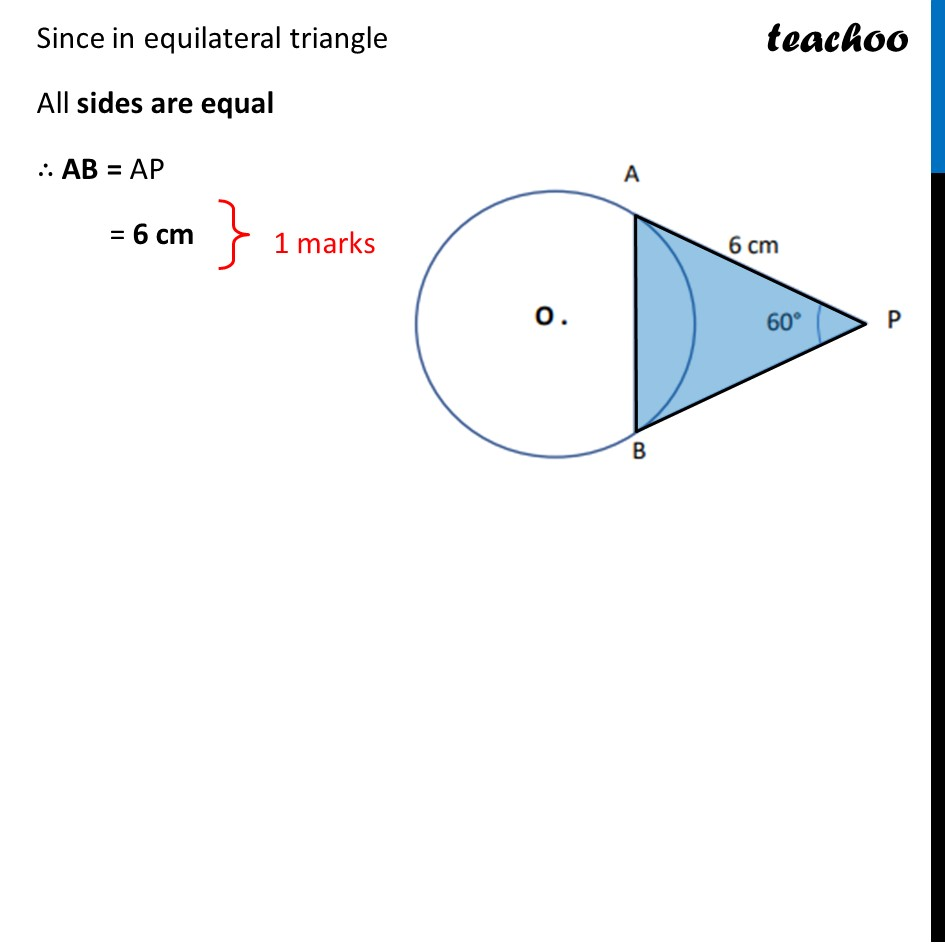CBSE Class 10 Sample Paper for 2022 Boards - Maths Basic [Term 2]

Class 10
Solutions of Sample Papers for Class 10 Boards

## PA and PB are the tangents drawn to a circle with centre O. If PA= 6 cm and  APB = 600 , then find the length of the chord AB.Learn in your speed, with individual attention - Teachoo Maths 1-on-1 Class

### Transcript

PA and PB are the tangents drawn to a circle with centre O. If PA= 6 cm and  APB = 600 , then find the length of the chord AB. We know that Tangent from external point are equal ∴ PA = PB Since angles opposite equal sides are equal Thus, ∠ PAB = ∠ PBA By Angle Sum property in Δ APB ∠ APB + ∠ PAB + ∠ PBA = 180° 60° + ∠ PAB + ∠ PAB = 180° 60° + 2∠ PAB = 180° 2∠ PAB = 180° − 60° 2∠ PAB = 120° ∠ PAB = (120° )/2 ∠ PAB = 60° Thus, in Δ APB ∠ PAB = ∠ PBA = 60° And, ∠ APB = 60° Since all angles are 60°, ∴ Δ APB is an equilateral triangle 60° + ∠ PAB + ∠ PAB = 180° 60° + 2∠ PAB = 180° 2∠ PAB = 180° − 60° 2∠ PAB = 120° ∠ PAB = (120° )/2 ∠ PAB = 60° Thus, in Δ APB ∠ PAB = ∠ PBA = 60° And, ∠ APB = 60° Since all angles are 60°, ∴ Δ APB is an equilateral triangle Since in equilateral triangle All sides are equal ∴ AB = AP = 6 cm